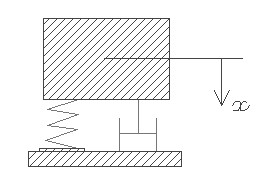FRVDMP,frq,dmr,dis,vel,sel
Free vibration with damping

frq         natural vibration frequency in KHz
dmr       damping ratio
dis         initial displacement from stable position in µm
vel         initial velocity in mm/s
sel         number denoting the selected result.
Use 1 for damped frequency of vibration, 2 for settling time and 3 for period of vibrationNotes

The natural frequency of vibration of structures estimated under Vibration > Free vibration assumes that damping is not present. In reality, some form of damping is always present in MEMS devices in the form of air damping or internal dissipation of energy of vibration. Damping will reduce the oscillation amplitude with every cycle and finally bring the structure to a stable state.

A vibration system with damping is usually modeled as shown in the picture above where the movement of the mass is resisted by the spring and the damper. The mass may leave the steady state with an instantaneous velocity, like that caused by a shock load, or the mass may be pushed to a new position and released with zero initial velocity or it could be a combination of both. No external force is acting on the mass.

This design interface can be used to estimate the vibration frequency, settling time and period of vibration considering the damping involved. The damping has significant effect on the vibration frequency depending on the damping ratio. The free vibration frequency is as estimated for the various structures under Vibration > Free vibration. If the damping ratio for air damping is not available it could be estimated using the design forms under Vibration > Damping for a given air gap distance. The vibration frequency is estimated for the under damped case only as there is no oscillation of displacement for the over damped and critically damped cases. For the critically damped system, the damping ratio is equal to one while for the over damped case it is greater than one and for under damped it is less than one. The period of vibration is the inverse of frequency in microseconds. Settling time is the time needed to bring the vibrating mass to a near stable position. Settling time is calculated as the time required to bring the displacement down to 0.1% of the displacement at 10 microseconds. This will be smaller for a critically damped part.

The plot shows displacement of the system over a time scale in microseconds for under damped, critically damped and over damped vibration based on the given damping ratio. For a critically damped system, the displaced mass will return to the steady state position immediately while for the over damped case it will take longer. The initial displacement is given as the first point of the plot in Y axis, and depending on the initial velocity it will have a phase shift. The displacement is plotted upto three time periods in the time scale. Using the crosshair tool, the displacement at any point after time t = 0 can be estimated from the graph. Settling time is the time in the X axis when the graph nearly flattens out at x = 0.

Assumptions

-There is no external force acting on the system.
-For air damping, the damping ratio should correspond to that particular mode of vibration.